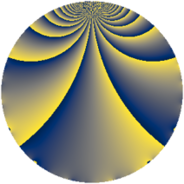# Properties

 Label 3024.1.ddLevel $3024$ Weight $1$ Character orbit 3024.dd Rep. character $\chi_{3024}(1423,\cdot)$ Character field $\Q(\zeta_{6})$ Dimension $4$ Newform subspaces $1$ Sturm bound $576$ Trace bound $0$

# Related objects

## Defining parameters

 Level: $$N$$ $$=$$ $$3024 = 2^{4} \cdot 3^{3} \cdot 7$$ Weight: $$k$$ $$=$$ $$1$$ Character orbit: $$[\chi]$$ $$=$$ 3024.dd (of order $$6$$ and degree $$2$$) Character conductor: $$\operatorname{cond}(\chi)$$ $$=$$ $$252$$ Character field: $$\Q(\zeta_{6})$$ Newform subspaces: $$1$$ Sturm bound: $$576$$ Trace bound: $$0$$

## Dimensions

The following table gives the dimensions of various subspaces of $$M_{1}(3024, [\chi])$$.

Total New Old
Modular forms 84 4 80
Cusp forms 12 4 8
Eisenstein series 72 0 72

The following table gives the dimensions of subspaces with specified projective image type.

$$D_n$$ $$A_4$$ $$S_4$$ $$A_5$$
Dimension 0 4 0 0

## Trace form

 $$4q + 4q^{5} + O(q^{10})$$ $$4q + 4q^{5} + 2q^{13} + 2q^{17} - 2q^{29} + 2q^{37} + 2q^{41} + 2q^{49} - 2q^{53} + 2q^{65} - 2q^{73} + 2q^{77} + 2q^{85} + 2q^{89} + 2q^{97} + O(q^{100})$$

## Decomposition of $$S_{1}^{\mathrm{new}}(3024, [\chi])$$ into newform subspaces

Label Dim. $$A$$ Field Image CM RM Traces $q$-expansion
$$a_2$$ $$a_3$$ $$a_5$$ $$a_7$$
3024.1.dd.a $$4$$ $$1.509$$ $$\Q(\zeta_{12})$$ $$A_{4}$$ None None $$0$$ $$0$$ $$4$$ $$0$$ $$q+q^{5}+\zeta_{12}^{5}q^{7}-\zeta_{12}^{3}q^{11}+\zeta_{12}^{2}q^{13}+\cdots$$

## Decomposition of $$S_{1}^{\mathrm{old}}(3024, [\chi])$$ into lower level spaces

$$S_{1}^{\mathrm{old}}(3024, [\chi]) \cong$$ $$S_{1}^{\mathrm{new}}(1008, [\chi])$$$$^{\oplus 2}$$